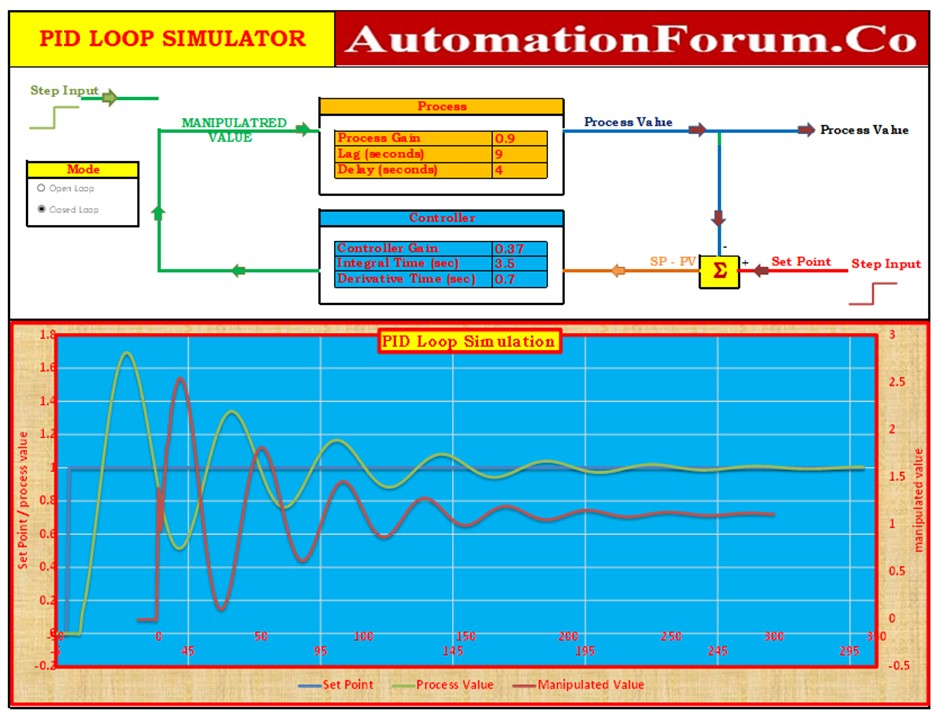# Excel based PID Loop Simulator• Excel based PID simulator is a tool developed in Microsoft Excel that enables users to simulate and analyze proportional-integral-derivative (PID) control systems. It allows for the modeling and testing of PID controllers, which are widely used in industrial automation and process control.
• The simulator provides a user-friendly interface where users can input system parameters, set control gains, and observe the system’s response to different inputs.
• It calculates the control signal based on the PID algorithm, updates the system’s output, and displays relevant graphs and data for analysis.
• Excel based PID simulator serves as a practical and accessible tool for educational purposes and preliminary evaluation of control strategies.
• A Proportional, Integral and Derivative (PID) controller on a First Order Time Delay (FOTPD) process can be simulated using the PID Loop Simulator, an Excel application.
• With the aid of this effective instrument, both open and closed loop processes can be mimicked.
• To learn the fundamentals of PID control and loop tuning, use this excellent resource.

## How to use

• Process Gain, Lag Time, and Delay Time are the three parameters that make up the First Order Time Delay (FOTPD) process. (Details regarding these factors are provided in the section below under “Process Model”).
• These settings should be specified in the Simulator’s “Process” section.
• In the “Controller” section, enter the controller values. The controller gain, integral time in seconds, and derivative time in seconds are these parameters.
• To examine the open loop response, select the “Open Loop” option.
• The controller is not utilized in this mode. The graph displays how the process value is affected by a step change in the controlled variable (such a control valve).
• The program replicates the PID equation in its parallel form, which is a common form in academic settings.
• The implemented equation is:

where:

t = Time

Manipulated Value (MV)

Controller Gain is K.

e = Controller Error = Process Value – Set Point

Integral Time = T i

Derivative Time: T d

## Excel file – PID Loop Simulator

You can obtain the Microsoft Excel spreadsheet for the PID simulator by using the URL that is provided below.

Scroll to Top